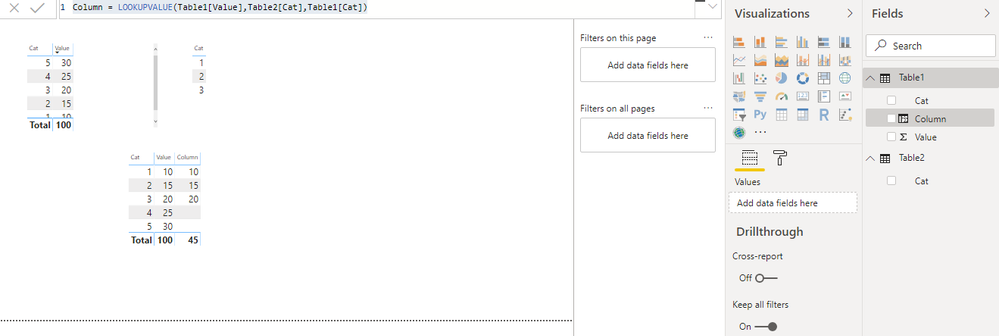cancel
Showing results for
Did you mean:Frequent Visitor

## Apply Filter with multiple values

Hello, I am trying to apply a filter on a table with values of another table column, I have tried this formula with no sucess :

Formula = sumx(CALCULATETABLE(Table1; Table1[Category] = DISTINCT(Table2[Cat])); Table1[Value])

My tables are :

Table 1

CategoryValue

 1 5 2 10 3 15 4 20 5 25

Table 2

Cat

 1 2 3
1 ACCEPTED SOLUTIONAnonymous
Not applicable

First I created a table for unique categories. go to modeling tab and click on new table

``Categories = VALUES('Table'[Category])``

then create a measure

``````Measure =
VAR _selectedCategories = VALUES(Categories[Category])
RETURN CALCULATE(SUM('Table'[Value]),FILTER('Table','Table'[Category] IN _selectedCategories))``````

Using category value from categories table in slicer and based on the selected values returning sum.

If it solved your purpose mark it as solved.

2 REPLIES 2Anonymous
Not applicable

First I created a table for unique categories. go to modeling tab and click on new table

``Categories = VALUES('Table'[Category])``

then create a measure

``````Measure =
VAR _selectedCategories = VALUES(Categories[Category])
RETURN CALCULATE(SUM('Table'[Value]),FILTER('Table','Table'[Category] IN _selectedCategories))``````

Using category value from categories table in slicer and based on the selected values returning sum.

If it solved your purpose mark it as solved.Anonymous
Not applicable
Column = LOOKUPVALUE(Table1[Value],Table2[Cat],Table1[Cat])

Create new measure on it to sum the values.